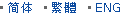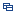| Recent Visit History | Join Audited Suppliers | Help | Region Guide
» » » » Nanjing Product List

256.

## Magnifier Double Convex Glass Lens[Nov 20, 2020]

Double Convex lens has a positive focal length, and with two outward surfaces. The two surfaces are with same radius of curvature, thereby minimizing spherical aberration. Some ...

257.

## Factory Customized Double Convex Lens[Nov 20, 2020]

Double Convex lens has a positive focal length, and with two outward surfaces. The two surfaces are with same radius of curvature, thereby minimizing spherical aberration. Some ...

258.

## Factory Optical Glass Bk7 Double Convex Lens[Nov 20, 2020]

Double Convex lens has a positive focal length, and with two outward surfaces. The two surfaces are with same radius of curvature, thereby minimizing spherical aberration. Some ...

259.

## Factory Custom Made Double Convex Lens[Nov 20, 2020]

Double Convex lens has a positive focal length, and with two outward surfaces. The two surfaces are with same radius of curvature, thereby minimizing spherical aberration. Some ...

260.

## Manufacturer Optical Glass Bk7 Double Convex Lens[Nov 20, 2020]

Double Convex lens has a positive focal length, and with two outward surfaces. The two surfaces are with same radius of curvature, thereby minimizing spherical aberration. Some ...

261.

## Focusing Glass Lens Bi Convex[Nov 20, 2020]

Bi Convex lens has a positive focal length, and with two outward surfaces. The two surfaces are with same radius of curvature, thereby minimizing spherical aberration. Some ...

262.

## Sapphire Glass Double Convex Lens[Nov 20, 2020]

Double convex lenses comprise two convex spherical surfaces, generally with the same radius of curvature. Bi convex lenses are used as magnifiers, condensing lenses and ...

263.

## Biconvex Lens for Visible Wavelength[Nov 20, 2020]

Bi Convex lens has a positive focal length, and with two outward surfaces. The two surfaces are with same radius of curvature, thereby minimizing spherical aberration. Some ...

264.

## Silicon Glass Double Convex Lens[Nov 20, 2020]

Double convex lenses comprise two convex spherical surfaces, generally with the same radius of curvature. Bi convex lenses are used as magnifiers, condensing lenses and ...

265.

## Large Size Bi Convex Lens[Nov 20, 2020]

Bi Convex lens has a positive focal length, and with two outward surfaces. The two surfaces are with same radius of curvature, thereby minimizing spherical aberration. Some ...

266.

## Calcium Fluoride Double Convex Lens[Nov 20, 2020]

Double convex lenses comprise two convex spherical surfaces, generally with the same radius of curvature. Bi convex lenses are used as magnifiers, condensing lenses and ...

267.

## Optical Glass Meniscus Lens for Binocular[Nov 20, 2020]

Meniscus lenses have one concave and one convex surface. For this lens type, the two curvatures are chosen to minimize the spherical aberration at one laser wavelength, hence ...

268.

## Optics Lens Optical Element Lens[Nov 20, 2020]

Bi Convex lens has a positive focal length, and with two outward surfaces. The two surfaces are with same radius of curvature, thereby minimizing spherical aberration. Some ...

269.

## Double Convex Lens-Optical Glass Bk7/Jgs1-Biconvex Lens[Nov 20, 2020]

Double convex lenses comprise two convex spherical surfaces, generally with the same radius of curvature. Bi convex lenses are used as magnifiers, condensing lenses and ...

270.

## Objective Optical Glass Bi Convex Lens[Nov 20, 2020]

Bi Convex lens has a positive focal length, and with two outward surfaces. The two surfaces are with same radius of curvature, thereby minimizing spherical aberration. Some ...

Hot Region Index: ABCDEFGHIJKLMNPQRSTUWXYZ